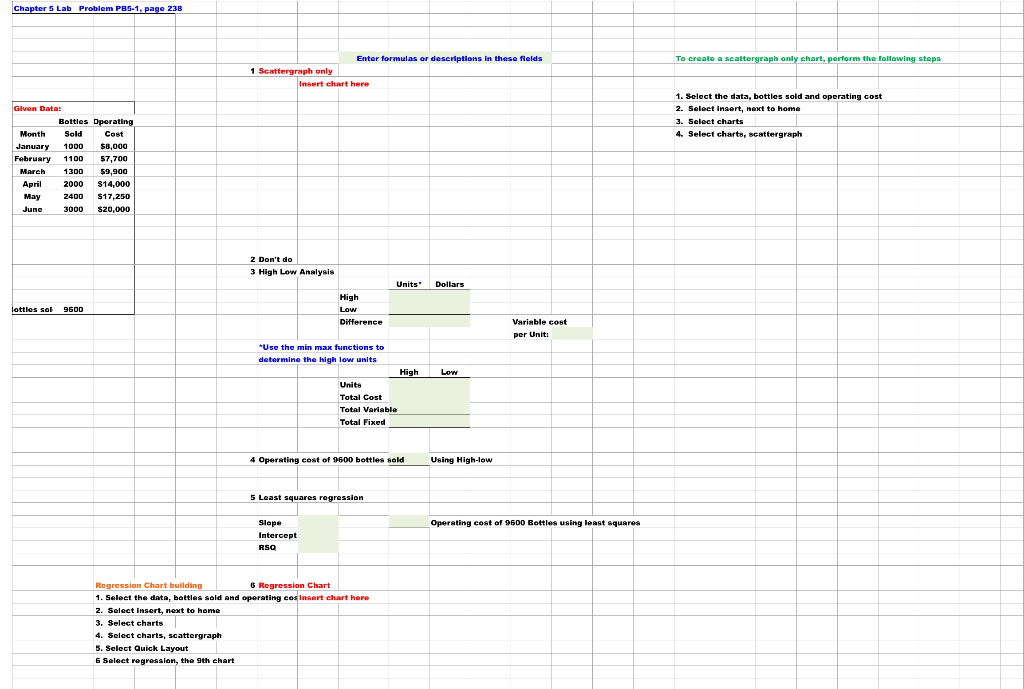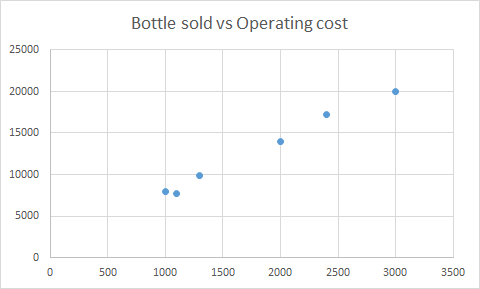Homework Help Question & Answers

5 Lab Problem PB5-1, page 238 the following step Enter formulas or descriptions in these fields T...5 Lab Problem PB5-1, page 238 the following step Enter formulas or descriptions in these fields To create a seattergraph only cha Insert chart here 1. Sclect the data, bottles sold and operating cost 2. Seloct insert, next to home Bottles Operating MonthSold Cost 4. Select charts, scattergraph January 1000 \$8,000 February 1100 57,700 Mareh 300 \$9,900 Apri 2000 S14,000 May 2400 517,250 3000 S20,000 3 High Low Analysis Units Dollars High Difference Variable cost per Unit Use the min max functions to determine th๑ high low units High Low Units Total Cost Total Fixed 4 Operating cost of 9600 bottles sold Using High-low 5 Least squares Operating cost of 9600 Bottles using least squares Intercept RSO Regression Chart buil 1. Select the data, bottles sold and operating coslnsert chart here 2. Select insert, next to homo 3. Select charts 4. Select charts, scattergraph .Select Quick Layout Regression Chart

Scatter plotUnits Dollars High 3000 20000 Low 1000 7700 Difference 2000 12300

The regression equation is
Operating cost = 1399 + 6.339 Bottles sold

Slope = 6.339

Intercept = 1399

With 0 bottles sold, operating cost = 1399

 Fixed Cost Variable cost Cost/Bottle January 1399 6601 6.60 February 1399 6301 5.73 March 1399 8501 6.54 April 1399 12601 6.30 May 1399 15851 6.60 June 1399 18601 6.20
 High Low Units 3000 1000 Total Cost 20000 7700 Total Variable 18601 6301 Total fixed 1399 1399
Add Answer of: 5 Lab Problem PB5-1, page 238 the following step Enter formulas or descriptions in these fields T...
More Homework Help Questions Additional questions in this topic.

• Step 1 = 3 boxes Step 2 = 6 boxes Step 3 = 11 boxes Step 4 = 18 boxes How many boxes at step 50

Need Online Homework Help?

Get FREE EXPERT Answers
WITHIN MINUTES
Related Questions